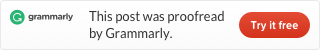Categories

# LeetCode 349 Intersection of Two Arrays [sort] java

Given two arrays, write a function to compute their intersection.

Example 1:

```Input: nums1 = [1,2,2,1], nums2 = [2,2]
Output: ```

Example 2:

```Input: nums1 = [4,9,5], nums2 = [9,4,9,8,4]
Output: [9,4]```

Note:

• Each element in the result must be unique.
• The result can be in any order.

We use one hashmap to store which number appears in the first array. And if any number in the second array is also in the hashmap 1. We put it to hashmap 2. In the end, we output every element in hashmap 2.